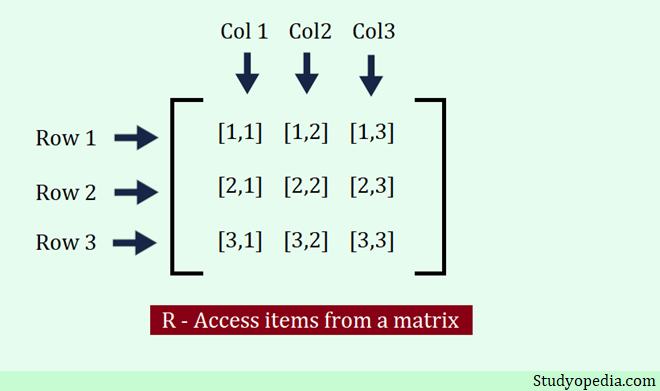## 14 Feb R Matrices

A matrix is an arrangement of data in two-dimensional form. It has rows and columns. In this lesson, we will learn how to create a Matrix and work on some operations on R Matrices:

• Representation of a Matrix
• Create a Matrix
• Iterate a Matrix
• Get the Matrix length
• Access Matrix Items
• Find the number of rows and columns in a Matrix

## Representation of a Matrix

To begin with, in the below figure, we have shown the representation of a matrix in the form of rows and columns. A row has a horizontal form whereas columns have a vertical form. It is a 3×3 matrix i.e. 3 rows and 3 columns:## Create a Matrix

To create a matrix in the R programming language, use the matrix() method. Using the nrow and ncol parameters, you need to set the number of rows and columns. In the below example, we have created a matrix. The c() is used to merge and concatenate the elements.

Let us see an example to create a matrix in the R programming language:

Output

## Iterate a Matrix

To iterate a matrix in the R programming language, use the for loop. Let us see an example:

Output

## Matrix Length

The length() function is used to in the R programming language to get the matrix length. Let us see an example:

Output

## Access Matrix Items

Use the [ ] brackets in R to access the list items i.e. elements of a List. Two values need to be set as a parameter. The 1st is the row position and the 2nd position is a column. Just set the position numbers of the rows and columns for the element you want to fetch as in the below figure:Let us see an example to access items from a matrix. We have access to all the items of a matrix to make the concept clear:

Output

## Find the number of rows and columns in a Matrix

The dim() function is used in the R programming language to find the number of rows and columns in a matrix. In the below example, we have a 3×3 matrix i.e. a matrix with 3 rows and 3 columns.

Let us see the example to find the count of rows and columns in an R matrix:

Output

If you liked the tutorial, spread the word and share the link and our website Studyopedia with others.